# Coils of transformer

The primary coil of the transformer has 1100 turns and is connected to a voltage of 220V. How many turns does the secondary coil have when the voltage on it is 55 V? Find the transformation ratio and decide what kind of transformation.

n2 =  275
k =  0.25

### Step-by-step explanation: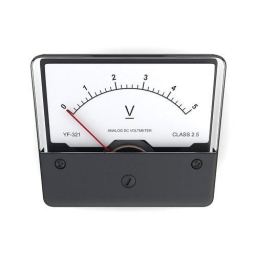We will be pleased if You send us any improvements to this math problem. Thank you!## Related math problems and questions:

• The transformer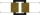The primary coil of the transformer has 1100 turns and is connected to a voltage of 220 V. How many turns does the secondary coil have when it has a voltage of 55 V? Determine the transformation ratio and decide what the transformation is.
• Coils of transformerWhat voltage is generated on the secondary coil of the transformer, which has 1500 turns, if the primary coil is connected to a voltage of 120 V and has 500 turns? What is the transformation ratio and what is the transformation about?
• Coils of transformerThe primary coil of the transformer has 400 turns, a current of 1.5 A passes through it and is connected to a voltage of 220 V. For the secondary coil, find the voltage, current, and a number of turns if the transformation ratio k = 0.1.
• The outputThe output voltage of the transformer is 880 V. The secondary coil has 1200 turns. Find the voltage to which the primary coil is connected and how many turns it has if a current of 1 A flows through it. Transformation ratio k = 4. What current flows throu
• TransformerTransformer - U1 = 230 V, N1 = 300, N2 = 1,200, I1 = 4 A. Calculate the transformation ratio, voltage and current in the secondary coil.
• Two resistorsTwo resistors 20 Ω and 60 Ω are connected in series and an external voltage of 400 V is connected to them. What are the electrical voltages on the respective resistors? Please comment!
• Filament of bulbThe filament of bulb has a 1 ohm resistivity and is connected to a voltage 220 V. How much electric charge will pass through the fiber when the electric current passes for 10 seconds?
• The bulbWhat is the resistance of a filament lamp when it is connected to a 9V battery and has a current of 120 mA?
• Voltmeter range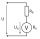We have a voltmeter which in the original set measures voltage to 10V. Calculate the size of the ballast resistor for this voltmeter, if we want to measure the voltage up to 50V. Voltmeter's internal resistance is 2 kiloohm/Volt.
• The bulbsThe bulbs are connected serially two bulbs and one resistor. Each bulb has a resistivity of 100 ohms and resistor of 60 ohms The voltage source in the circuit is 24 volts. Do you know what voltage to measure on individual appliances?
• Mains voltageHow much electric current flows through the appliance with a resistance of 40kΩ, which is connected to the mains voltage (230 V)?
• TransformerSolve the textbook problems - transformer: a) N1 = 40, N2 = 80, U2 = 80 V, U1 =? b) N1 = 400, U1 = 200 V, U2 = 50 V, N2 =?
• Current in the conductorCalculate the current in the conductor (in mA) if it is connected to a 4.5 V voltage source and its resistance is 20 (ohm).
• Lowest voltage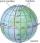Three resistors with resistors R1 = 10 kΩ, R2 = 20 kΩ, R3 = 30 kΩ are connected in series and an external voltage U = 30 V is connected to them. On which resistor is the lowest voltage?
• Electric input powerSolve problems related to electric power: a) U = 120 V, I = 0.5 A, P =? b) P = 200 W, U = 230 V, I =? c) I = 5 A, P = 2200W, U =?
• Bicycle gear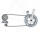The pedal bicycle wheel has 56 teeth and a rear 20 gear tooth. How many times does the bicycle wheel turn when you make 20 turns of the pedal wheel?
• Coil as a girl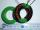The electrical resistance of the copper wire coil is 2.0 ohms. What current runs through the coil when the voltage between the terminals is 3.0 V?